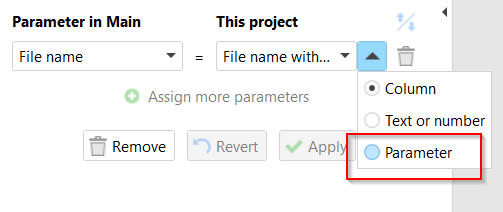# How to assign values in columns to a parameter?

Is there a way to iterate through a table and assign the value in each row to a different parameter? There will always be 3 rows and I want to assign a parameter that is assigned based on the value in column A. “If column A = 1 then Assign parameter Fruit to the value in Column B, else if column a = 2 then assign parameter Veggie in column B, else Carbs”. How could I do this?
Column A ----Column B
1 Fruit
2 Veggie
3 Carbs

Hi @MorphWizard and welcome to the Community!

The idiomatic way would be to precalculate the correct values in columns before iteration. In this case, when you do the iteration you have the correct values assigned.

In your case, you can calculate [Column B] as

``````[Column B] = IF [A] = 1 THEN {Fruit} ELSE IF [A]=2 THEN {Veggie} ELSE "Carbs"
``````

Or, even better, use the “Rule” action that was specifically designed to deal with nested conditional calculations:

1 Like

InterestingThanks for helping, but that didn’t work the way I wanted it to. Instead, how would I iterate “for each row in table, assign a parameter to each column and perform Action A”? For example, Column A will have a parameter assigned {Number}, Column B will have a parameter assigned {Food} and then I have 3 rows in the table. I want to perform the same action for each row. How would I do that?

I’m not sure I understand. How is it different from a regular iteration done with the “Iterate” action?

You can assign parameters to columns before iteration using expressions, or even simpler, you can assign parameters with parameters right in the “Iterate” action: